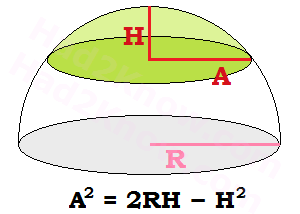# Surface Area and Volume of a Spherical Cap

Spherical Cap Calculator
Cap Height H =
Radius    of cap:          A =
of sphere:   R =In spherical geometry, a cap is section of a sphere obtained by slicing it with a plane. A spherical cap has a circular base and a dome that is flatter than a hemispherical dome. (A hemisphere is spherical cap obtained by cutting the sphere in half.)

You can figure the volume and surface area of a spherical cap if know its height and either the radius of its base or the radius of the larger sphere. The calculator below will compute the surface area and volume if you input the height H, and either the base radius A or sphere radius R. You can also apply the formulas described below.

### Volume Formula for a Spherical Cap

The equation that relates A, R, and H is

A2 = 2RH - H2

To find the volume in terms of A and H, the equation is

Volume = (pi/6)H(3A2 + H2)

To find the volume in terms of R and H, the equation is

Volume = (pi/3)H2(3R - H)

(Pi is the mathematical constant 3.14159265358...)

### Surface Area Formula for a Spherical Cap

The surface area formula excluding the base is

Surface Area = (pi)(A2 + H2), or
Surface Area = 2(pi)RH.

The formula for its total surface area (including the base of the cap) is

Surface Area = (pi)(A2 + H2) + (pi)A2, or
Surface Area = 2(pi)RH + (pi)(2RH - H2).

### Example

Suppose a spherical cap is 25 cm tall and the radius of the base is 35 cm. So we have H = 25, A = 35, and R = 37. The volume is

(pi/3)(252)(3(37) - 25) = 56,287 cm3

The surface area (excluding the base) is

2(pi)(37)(25) = 5,812 cm2